# Surface and volume

Calculate the surface and volume of a cylinder whose height is 8 dm and the radius of the base circle is 2 dm

S =  125.6637 dm2
V =  100.531 dm3

### Step-by-step explanation: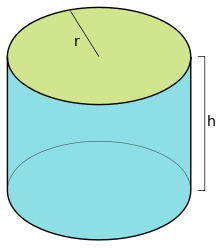Did you find an error or inaccuracy? Feel free to write us. Thank you!Tips to related online calculators
Tip: Our volume units converter will help you with the conversion of volume units.

## Related math problems and questions:

• Cylinder surface areaVolume of a cylinder whose height is equal to the radius of the base is 678.5 dm3. Calculate its surface area.
• Cylinder - h2Cylinder volume is 2.6 liters. Base area is 1.3 dm2. Calculate the height of the cylinder.
• Curved surface area CSA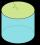A cylinder 5cm high has a base radius(7/2) cm. Calculate the curved surface area.
• Calculate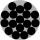Calculate the area of a circle whose radius is 5 dm.
• Rotating coneThe rotating cone has a base diameter of 18 dm and a height of 12 dm. Calculate the volume V.
• Hexagonal prism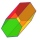Calculate the volume and surface of a regular hexagonal prism with the edge of the base a = 6 cm with the corresponding height v1 = 5.2cm and the height of the prism h = 1 dm.
• Height of the cylinderThe cylinder volume is 150 dm cubic, the base diameter is 100 cm. What is the height of the cylinder?
• Surface and volumeFind the surface and volume of a cuboid whose dimensions are 1 m, 50 cm, and 6 dm.
• Calculate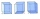Calculate the surface of a regular quadrilateral prism whose base edge is 2.4dm and the height of the prism is 38cm.
• Prism 4 sidesFind the surface area and volume four-sided prism high 10cm if its base is a rectangle measuring 8 cm and 1.2dm
• Cube V2SThe volume of the cube is 27 dm cubic. Calculate the surface of the cube.
• Surface areaCalculate the surface area of a four-sides 2-m high prism which base is a rectangle with sides 17 cm and 1.3 dm
• The volumeThe volume of a solid cylinder is 260 cm3 the cylinder is melt down into a cuboid, whose base is a square of 5cm, calculate the height of the cuboid and the surface area of the cuboid
• CylinderThe cylinder surface is 922 dm2. Its height is equal to the radius of the base. Calculate the height of this cylinder.
• Cylinder heightCalculate the height of the cylinder and its surface is 2500 dm2 and the bases have a diameter 5dm.
• Trapezoidal prism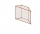Calculate the volume of a prism with a trapezoidal base with side a = 6dm, side c = 4 dm, height of the prism = 8dm. The height of the trapezoid is va = 3dm.
• Volume and surfaceCalculate the volume and surface area of the cylinder when the cylinder height and base diameter is in a ratio of 3:4 and the area of the cylinder jacket is 24 dm2.